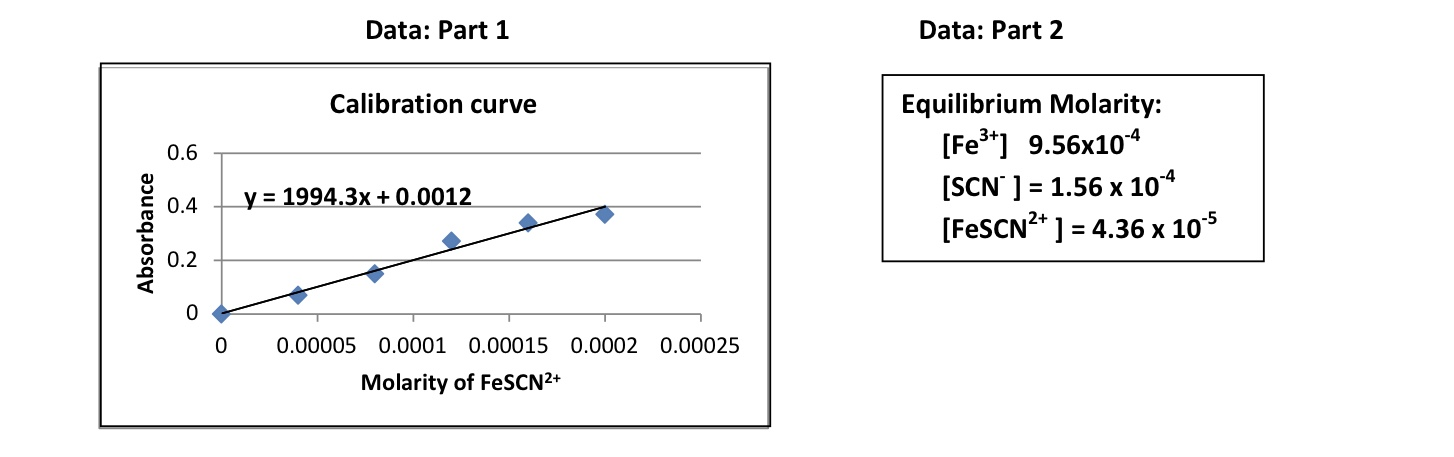# What was the equilibrium equation for the reaction given by the data above? What was the equilibrium expression for the reaction? The calibration curve shows a direct correlation between absorbance and concentration. What is the law that defines this relationship? If the absorbance value for the FeSCN tested in part 2 was 0.087, how was the equilibrium concentration determined using the information provided by the calibration curve? 28. What is the value for the equilibrium const